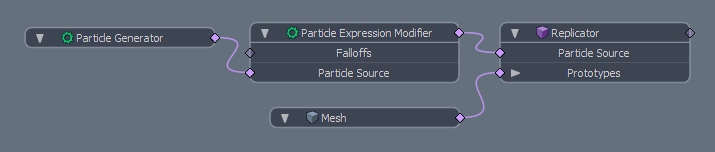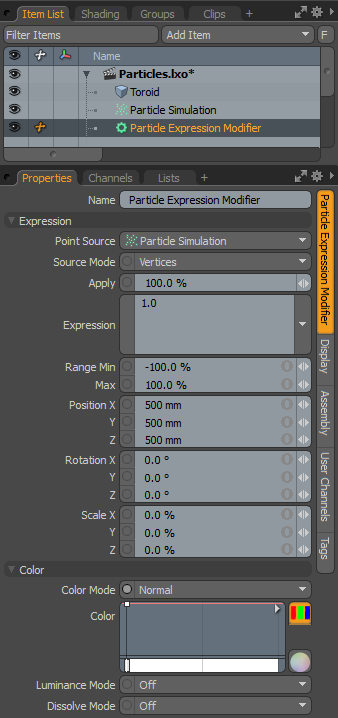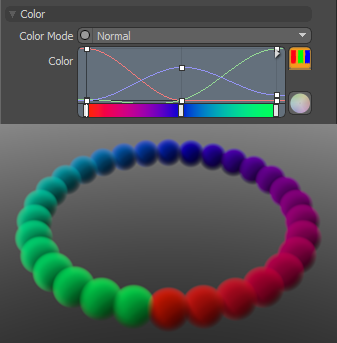# Particle Expression Modifier

The particle expression modifier allows you to apply expressions to particles. An expression is a set of instructions that are represented as a mathematical formula. In the case of this particle expression modifier, certain mathematical controls can be applied to particles to control various aspects of the particles motions or positions, or can assign a control when a certain condition is met. The expression itself controls the way in which the particles are affected, the Apply and Range options control the degree of its affect, and the transform options control the actual modification that is applied to the target.

#### Adding a Particle Expression Modifier

You can add a particle expression modifier item in the Setup interface under the Particles sub-tab, or by using the Add Item button of the Items List. Click the button to open the menu, and under Particles > Modifiers, click Particle Expression Modifier.

Once added to the scene, you need to drag the item itself from the Items list and drop it in the Schematic viewport. The expression modifier is connected between the point source element and the point target items, such as a Replicator. The connection should look like this image.The expression modifies the particle values from the source, which can be any number of particle items, either static or dynamic. In this case, it is a simple particle Generator. Those values are replaced with the expression evaluations and then passed on to the target. The values returned by the expression are multiplied by the values set in the other channels of the modifier. For example, for a sine wave on the Y axis that moves through the range of the particles with time, the expression is sin(T+PID). Where using PID (particle ID) is an offset to the current time applied to the Y position. Then you just need to set the Position Y transform option to a positive value, such as 1 m, and all the other Position, Rotation and Scale channels to 0.0. When the Timeline is played, the sine wave transforms the positions of the particles 1 m modulating up and down with the default Range Min/Max values of 100%/100%, respectively.

When the particle expression modifier is selected, the following attributes appear in the Properties panel.Option

Description

Name

Displays the current item name. To change it, click on the field and type in the new name.

Expression

Point Source

Defines the source of the particles that are affected by the expression modifier.

Source Mode

Specifies the way the particle modifier affects the source. Vertices uses the point positions, Polygons uses the polygon centers, and Detached Vertices uses the point positions of vertices that are not part of polygons.

Apply

Determines the overall degree that the expression affects the target. At 0% the target is not affected at all, at 100% the target is fully affected. This allows you to transition to the modified state.

Formula

This is where the actual expression formula is entered.

Range Min/Max

Determines the amount of transform applied to the target over the entire range of the particles acting as a multiplier on the value. For example, if the Scale is set to 200% on all three axes, and the Range Min/Max is 100%/100% respectively, then the first particle would be scaled 200%, attenuating the value toward the last particle scaled at 200%. The range is determined in the order of the Particle ID.

Seed

The initial number used when generating the random procedural values. Different Seed values produce different random variations and can be useful in changing the result.

PositionX/Y/Z

Defines the position values added to the target for each axes.

RotationX/Y/Z

Defines the rotation values added to the target for each axes.

Scale X/Y/Z

Defines the scale values added to the target for each axes.

Color

Allows you to use mini gradient editors to assign and control different attributes for the resulting particles. These attributes are then passed along to the final effect, working with both volumes and sprites. The gradient values are generated sequentially, dividing the gradient itself into the number of generated particles it is assigned to, each receiving the value for that position along the gradient. For example, a red to blue gradient assigned to a linear array of particles produces one red particle at the start. The particle colors then fade toward the blue color in even increments along the length of the array.

There are three different settings that can be applied to their associated surface attributes. Any of these functions are enabled by selecting any option other than Off (the default value that disabled the function). The selected Mode determines the blending of the gradient with the base setting of the target element.

Color Mode

Determines how the resulting gradient applies, defining the blending mode of the colors as they apply to the base particle coloring. The Color gradient editor that opens allows you to define the actual gradient values that are applied.

Luminance Mode

Determines how the resulting gradient applies, defining the blending mode of the luminosity value as it applies to the base particle luminosity. The Luminosity gradient editor that opens allows you to define the actual gradient values that are applied.

Dissolve Mode

Determines how the resulting gradient applies, defining the blending mode of the colors as they apply to the base particle transparency. The Dissolve gradient editor that opens allows you to define the actual gradient values that are determine the transparent amount.A Normal color mode gradient applied to a particle generator's radial array

#### Particle Variables

The following table lists per-particle variables supported by the parser.

Note:  For AGE, PATH, and MASS you need to enable these features in the Particle Simulation item, otherwise they return zero.

User channels can be referenced by name in the expression.

Variable

Meaning

PX, PY, PZ

Position for X, Y & Z

PPX, PPY, PPZ

Previous Position for X, Y & Z

RX, RY, RZ

Rotation for X, Y & Z

SX, SY, SZ

Scale for X, Y & Z

VX, VY, VZ

Velocity for X, Y & Z

FX, FY, FZ

Force for X, Y & Z

CR, CG, CB

Color for R, G & B

LUM

Luminance

PID

Particle ID

PCNT

Particle Count

AGE

Particle Age

MASS

Particle Mass

SIZE

Particle Size

PATH

The distance the particle has traveled

DISS

Dissolve

RATE

Rate

ITEM

Item

T

Time

#### Built-in Function

The following table gives an overview of the functions supported by the default implementation. It lists the function names, the number of arguments and a brief description.

Name

Meaning

sin

sine function

cos

cosine function

tan

tangens function

asin

arcus sine function

acos

arcus cosine function

atan

arcus tangens function

sinh

hyperbolic sine function

cosh

hyperbolic cosine

tanh

hyperbolic tangens function

asinh

hyperbolic arcus sine function

acosh

hyperbolic arcus tangens function

atanh

hyperbolic arcur tangens function

log2

logarithm to the base 2

log10

logarithm to the base 10

log

logarithm to the base 10

ln

logarithm to base e (2.71828...)

exp

e raised to the power of x

sqrt

square root of a value

sign

sign function -1 if x<0; 1 if x>0

rint

round to nearest integer

abs

absolute value

min

min of all arguments

max

max of all arguments

sum

sum of all arguments

avg

mean value of all arguments

mod(a,b)

returns the remainder of a divided by b

clamp(a, min, max)

given a value a, if a is less then min, min is returned. If greater then max, max is returned. If in between min and max, a is returned.

#### Built-in Binary Operators

The following table lists the default binary operators supported by the parser.

Operator

Meaning

Priority

=

assignment *

-1

&&

logical and

1

||

logical or

2

<=

less or equal

4

>=

greater or equal

4

!=

not equal

4

==

equal

4

>

greater than

4

<

less than

4

+

5

-

subtraction

5

*

multiplication

6

/

division

6

^

raise x to the power of y

7

#### Other Operators

muParser has built in support for the if/then/else operator. It uses lazy evaluation in order to make sure only the necessary branch of the expression is evaluated.

Operator

Meaning

Remarks

?:

if then else operator

C/C++ style syntax